# Creating FFTs for heart disease

#### 2018-09-15

The following example follows the tutorial presented in Phillips et al. (2017) FFTrees: A toolbox to create, visualize, and evaluate fast-and-frugal decision trees. available online at http://journal.sjdm.org/17/17217/jdm17217.pdf

## Step 1: Install and load the FFTrees package

You can install FFTrees from CRAN using install.packages() (you only need to do this once)

# Install the package from CRAN
install.packages("FFTrees")

To use the package, you first need to load it into your current R session. You can load the package using library()

# Load the package
library(FFTrees)

The package contains several guides (like this one). To open the main guide, run FFTrees.guide()

# Open the main package guide
FFTrees.guide() 

## Step 2: Create FFTs from training data (and test on testing data)

In this example, we will create FFTs from a heart disease data set. The training data are in an object called heart.train, and the testing data are in an object called heart.test. For these data, we will predict diagnosis, a binary criterion that indicates whether each patent has or does not have heart disease (i.e., is at high-risk or low-risk).

To create the FFTrees object, we’ll use the function FFTrees() with two main arguments: formula, a formula indicating the binary criterion as a function of one or more predictors to be considered for the tree (the shorthand formula = diagnosis ~ . means to include all predictors), and data, the training data.

# Create an FFTrees object

heart.fft <- FFTrees(formula = diagnosis ~ .,           # Criterion and (all) predictors
data = heart.train,                # Training data
data.test = heart.test,            # Testing data
main = "Heart Disease",            # General label
decision.labels = c("Low-Risk", "High-Risk"))  # Labels for decisions

The resulting trees, decisions, and accuracy statistics are now stored in the FFTrees object called heart.fft.

#### Other arguments

• algorithm: There are several different algorithms available to build FFTs, including “ifan” (Phillips et al. 2017), “dfan”(Phillips et al. 2017), “max” (Martignon, Katsikopoulos, and Woike 2008), and “zigzag” (Martignon, Katsikopoulos, and Woike 2008).
• max.levels: Changes the maximum number of levels allowed in the tree.

The following arguments apply to the “ifan” and “dfan” algorithms only:

• goal.chase: The goal.chase argument changes which statistic is maximized during tree construction (for the “ifan” and “dfan” algorithms only). Possible arguments include “acc”, “bacc”, “wacc”, “dprime”, and “cost”. The default is “wacc” with a sensitivity weight of 0.50 (which is identical to “bacc”)
• goal: The goal argument changes which statistic is maximized when selecting trees after construction (for the “ifan” and “dfan” algorithms only). Possible arguments include “acc”, “bacc”, “wacc”, “dprime”, and “cost”.
• my.tree: You can define a tree verbally as a sentence using the my.tree argument. See Defining an FFT verbally for examples.

## Step 3: Inspect and summarize FFTs

Now we can inspect and summarize the trees. We will start by printing the object to return basic information to the console:

heart.fft   # Print the object
## Heart Disease
## FFT 1 (of 7) predicts diagnosis using 3 cues: {thal, cp, ca}
##
##  If thal = {rd,fd}, decide High-Risk.
##  If cp != {a}, decide Low-Risk.
##  If ca <= 0, decide Low-Risk, otherwise, decide High-Risk.
##
##                    train   test
## cases       .n    150.00 153.00
## hits        .hi    54.00  64.00
## misses      .mi    12.00   9.00
## false al    .fa    18.00  19.00
## corr rej    .cr    66.00  61.00
## speed       .mcu    1.74   1.73
## frugality   .pci    0.88   0.88
## cost        .cost   0.20   0.18
## accuracy    .acc    0.80   0.82
## balanced    .bacc   0.80   0.82
## sensitivity .sens   0.82   0.88
## specificity .spec   0.79   0.76
##
## pars: algorithm = 'ifan', goal = 'wacc', goal.chase = 'wacc', sens.w = 0.5, max.levels = 4

The output tells us several pieces of information:

• The tree with the highest weighted sensitivity wacc with a sensitivity weight of 0.5 is selected as the best tree.
• The best tree, FFT #1 uses three cues: thal, cp, and ca.
• Several summary statistics for this tree in training and test data are then summarized.

To summarise performance statistics for a tree across training and test data, use the summary() function (use the tree = X argument to specify a diffent tree)

# Pring summary statistics of all trees
summary(heart.fft)
##        train    test
## n    150.000 153.000
## hi    54.000  64.000
## mi    12.000   9.000
## fa    18.000  19.000
## cr    66.000  61.000
## mcu    1.740   1.725
## pci    0.876   0.877
## cost   0.200   0.183
## acc    0.800   0.817
## bacc   0.802   0.820
## sens   0.818   0.877
## spec   0.786   0.762

All statistics can be derived from a 2 x 2 confusion table like the one below. For definitions of all accuracy statistics, look at the accuracy statistic definitions vignette.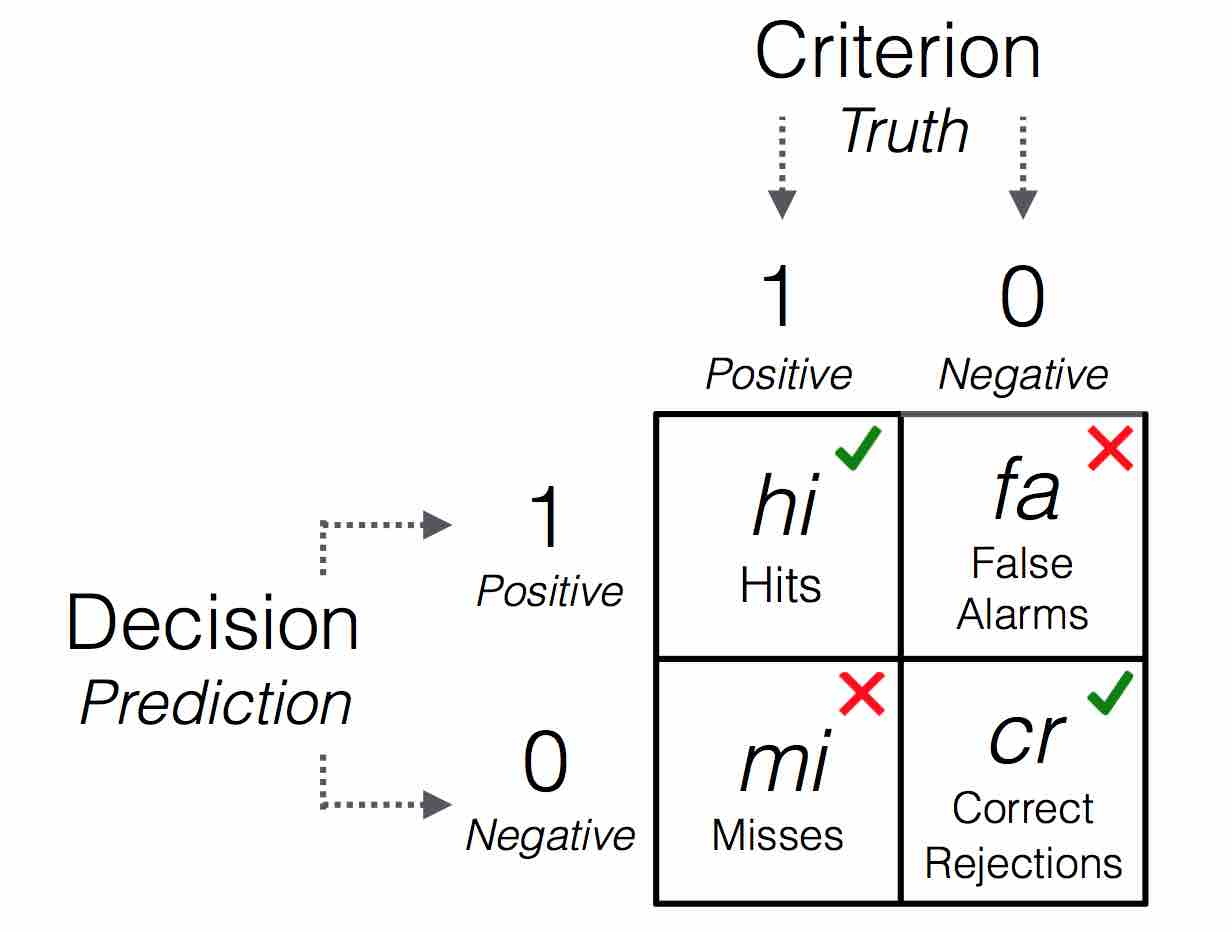Confusion table illustrating frequencies of 4 possible outcomes.

## Step 4: Visualise the final FFT

Plotting FFTrees

To visualize a tree, use plot():

# Plot the best FFT when applied to the test data

plot(heart.fft,              # An FFTrees object
data = "test")          # Which data to plot? "train" or "test"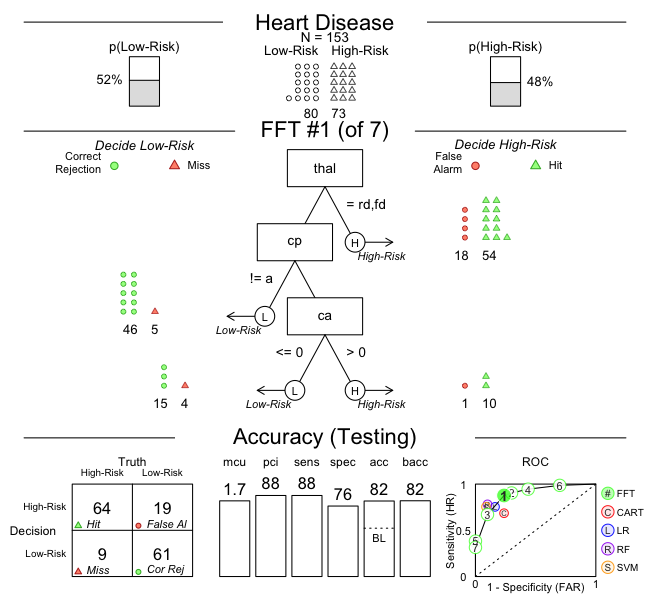#### Other arguments

• tree: Which tree in the object should beplotted? To plot a tree other than the best fitting tree (FFT #1), just specify another tree as an integer (e.g.; plot(heart.fft, tree = 2)).
• data: For which dataset should statistics be shown? Either data = "train" (the default), or data = "test"
• stats: Should accuracy statistics be shown with the tree? To show only the tree, without any performance statistics, include the argument stats = FALSE
# Plot only the tree without accuracy statistics
plot(heart.fft,
stats = FALSE)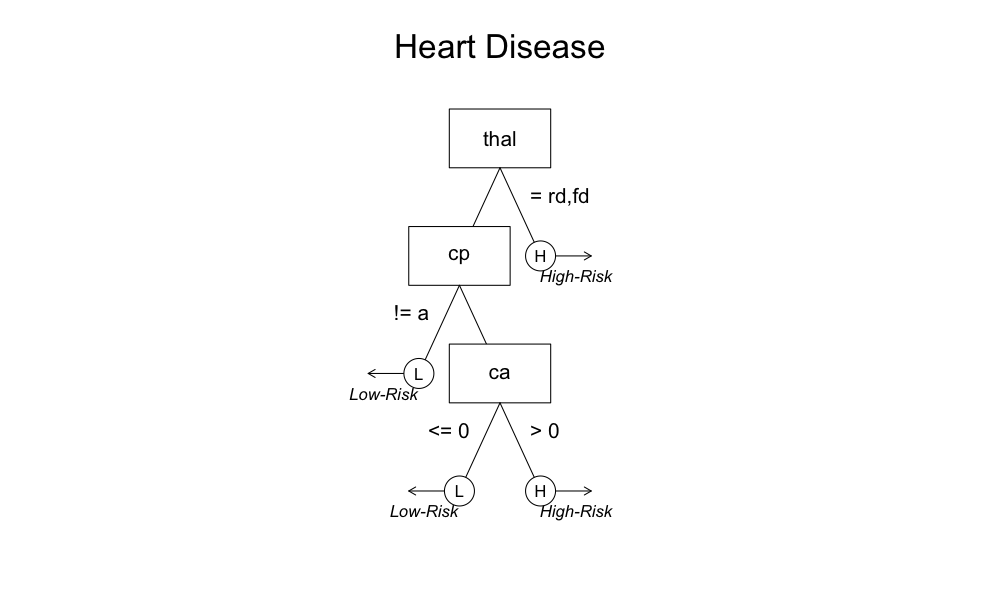• comp: Should statistics from competitive algorithms be shown in the ROC curve? To remove the performance statistics of competitive algorithms (e.g.; regression, random forests), include the argument comp = FALSE

• what: To show individual cue accuracies in ROC space, include the argument what = "cues":

# Show marginal cue accuracies in ROC space
plot(heart.fft,
what = "cues")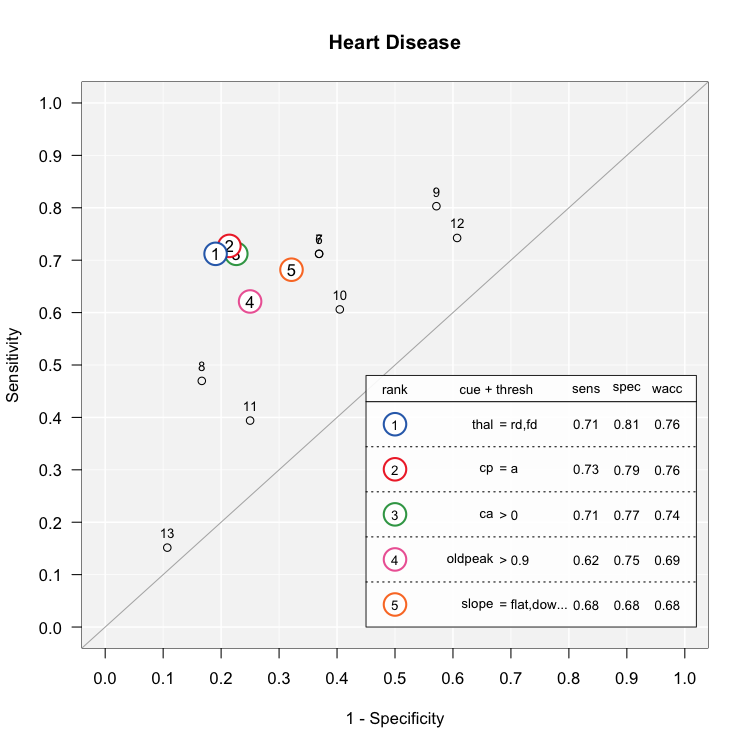### Accesing outputs

An FFTrees object contains many different outputs, to see them all, run names()

# Show the names of all of the outputs in heart.fft

names(heart.fft)
##   "formula"          "data.desc"        "cue.accuracies"
##   "tree.definitions" "tree.stats"       "cost"
##   "level.stats"      "decision"         "levelout"
##  "tree.max"         "inwords"          "params"
##  "comp"             "data"

Here is a brief description of each of the outputs:

Output Description
formula The formula used to generate the object
data.desc Descriptions of the original training and test data
cue.accuracies Cue thresholds and accuracies
tree.definitions Definitions of all trees, including cues, thresholds and exit directions
tree.stats Performance statistics for trees
cost Cost statistics for each case and tree.
level.stats Cumulative performance statistics for all trees.
decision Classification decisions
levelout The level at which each case is classified
tree.max The best performing training tree in the object.
inwords A verbal description of the trees.
auc Area under the curve statistics
params A list of parameters used in building the trees
comp Models and statistics for competitive algorithms (e.g.; regression, (non-frugal) decision trees, support vector machines)
data The original training and test data

### Predicting new data

To predict classifications for a new dataset, use the standard predict() function. For example, here’s how to predict the classifications for data in the heartdisease object (which actually is just a combination of heart.train and heart.test)

# Predict classifications for a new dataset
predict(heart.fft,
data = heartdisease)

### Defining an FFT in words

Defining an FFT verbally

If you want to define a specific FFT and apply that tree to data, you can define it using the my.tree argument.

# Create an FFT manuly
my.heart.fft <- FFTrees(formula = diagnosis ~.,
data = heart.train,
data.test = heart.test,
main = "My custom Heart Disease FFT",
my.tree = "If chol > 350, predict True.
If cp != {a}, predict False.
If age <= 35, predict False. Otherwise, predict True")

Here is the result (It’s actually not too bad, although the first node is pretty worthless)

plot(my.heart.fft)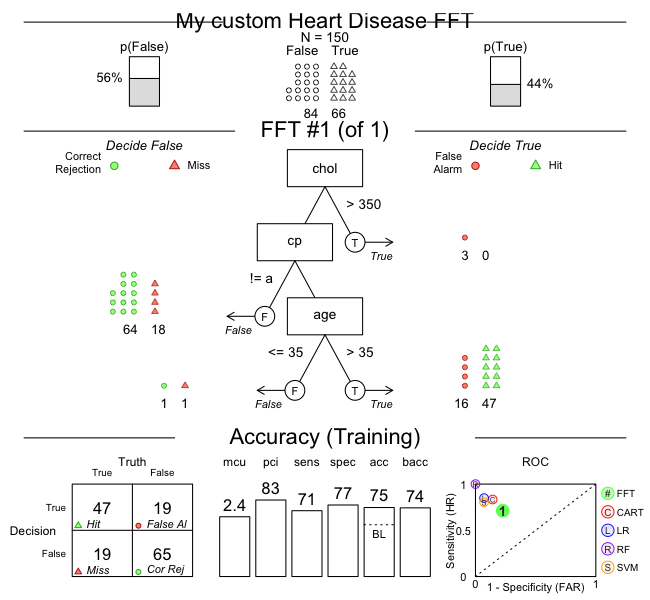### Create a forest of FFTs

The FFForest() function conducts a bootstrapped simulation on the training data, thus creating a forest of several FFTs. This can give you insight as to how important different cues are in the dataset

# Create an FFForest object (can take a few minutes)
heart.fff <- FFForest(formula = diagnosis ~.,
data = heartdisease,
ntree = 10,
train.p = .5)

Plotting the result shows cue importance and co-occurrence relationships:

plot(heart.fff)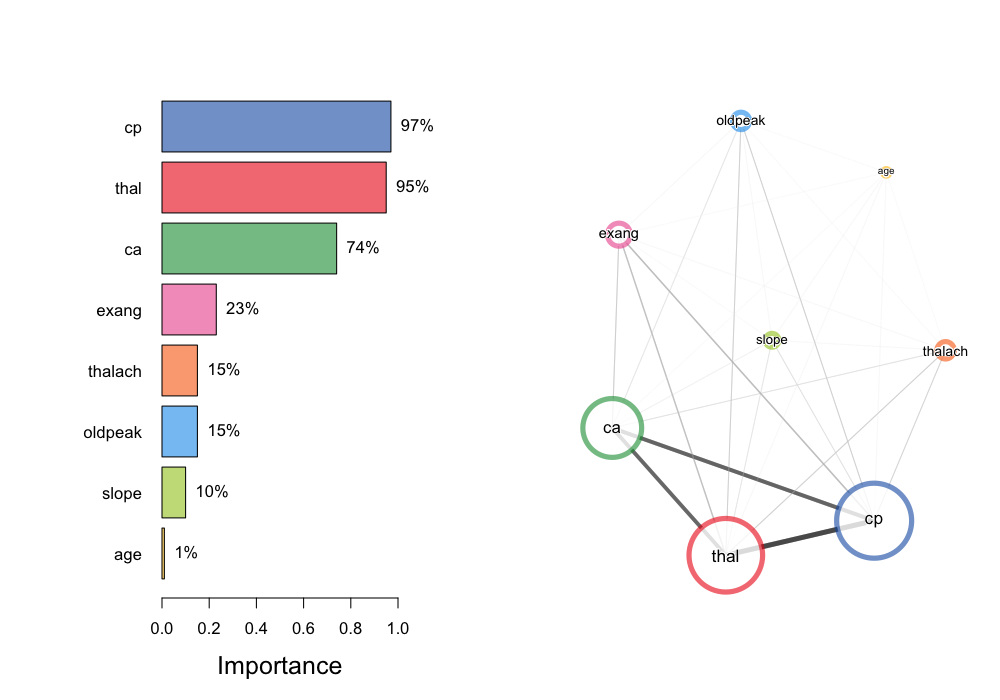Here, we see that the three cues cp, thal, and ca occur the most often in the forest and thus appear to be the most important three cues in the dataset.